# How to Make a Dollar with Coins

How to Make a Dollar with Coins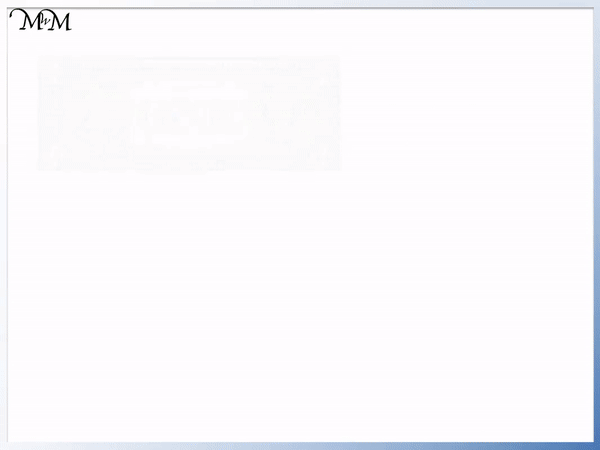• Here are some common combinations of ways to make a US dollar by making a total of 100 cents.
• A dollar is worth 100 cents and each dollar bill is the same value as 100 penny coins.
• Each dime is worth 10 cents so ten dimes make one dollar because 10 x 10 = 100 cents.
• Each nickel is worth 5 cents so twenty nickels make one dollar because 20 x 5 = 100 cents.
• Each quarter is worth 25 cents. 4 x 25 = 100 cents and so four quarters make one dollar.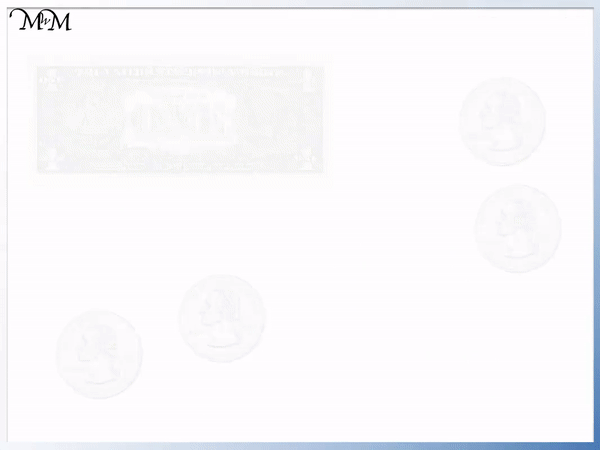• We are asked to count the American money shown.
• We have one US dollar bill.
• We have four quarters which is one of the combinations of coins that make an American dollar.
• We have a dollar bill plus another dollar from the four quarters.
• We have two dollars in total, which we can write as $2.#### Counting US Money Dollars and Cents: Interactive Question Generator #### Adding US Dollars and Cents Worksheets and Answers # How to Make an American Dollar using Cents, Nickels, Dimes and Quarters A dollar can be made from any combination of coins that add up to make 100 cents. Here are some common ways to make a dollar with change: • 100 pennies • 4 quarters • 10 dimes • 20 nickels • 3 quarters, 2 dimes and a nickel • 2 quarters and 5 dimes There are many other ways to make a dollar and we will look at some of the common ways below. Below is an American one dollar bill, which we commonly refer to as a dollar or US dollar. Each dollar is worth 100 cents and each dollar bill holds the same value as one hundred cents. A dollar is therefore the same worth as one hundred 1¢ coins combined. Instead of carrying one hundred 1¢ coins, we can use the one dollar note.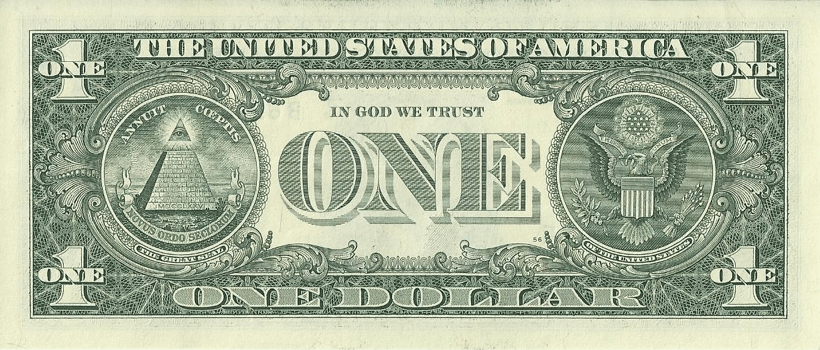The symbol for a dollar is ‘$’.

Since one dollar is worth 100 cents we can write:

$1 = 100¢.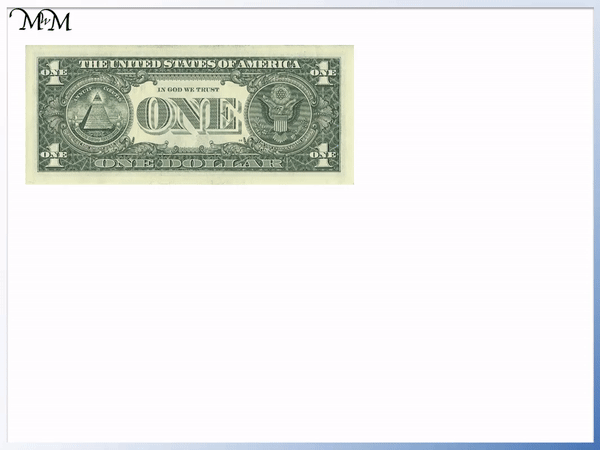There are many different ways that we can make$1. We can make a dollar by using any combination of US coins that add up to 100¢.

One way to make a dollar is to use 100 cent coins.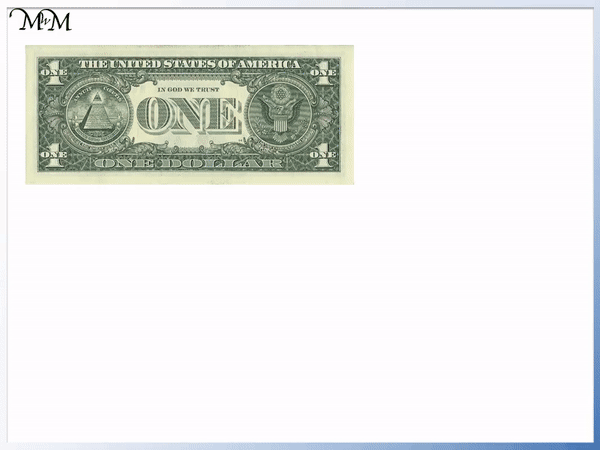Another way that we can make a dollar is with ten 10¢ coins (ten dimes).This is because each dime is worth ten cents:

10¢ + 10¢ + 10¢ + 10¢ + 10¢ + 10¢ + 10¢ + 10¢ + 10¢ + 10¢ = 100¢

10 x 10¢ = 100¢Another way to make a dollar is to use twenty nickel coins, which are each worth 5¢.This is because 20 x 5 = 100.20 x 5¢ = 100¢

100¢ = $1 Another way to make a dollar is with four quarter dollar coins. Each quarter is worth 25¢. The name ‘quarter’ helps us to remember that four quarters make a whole dollar.25¢ + 25¢ + 25¢ + 25¢ =$1

4 x 25¢ = 100¢

100¢ = $1# Examples of Counting Amounts of Money In the example below, we are asked to work out the total amount of money. We count any coins that we have into groups that make one dollar.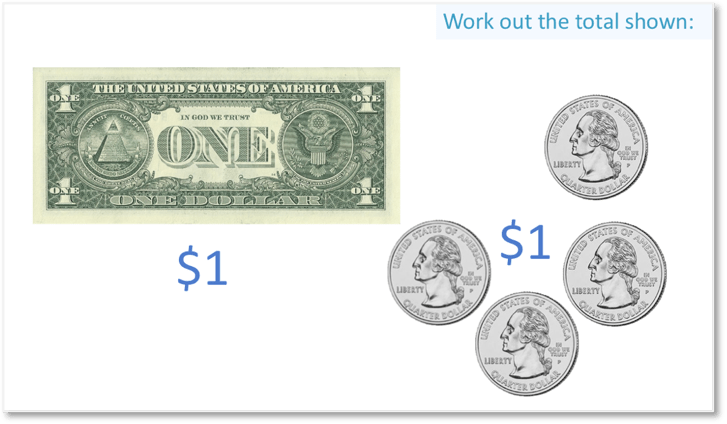We have a$1 bill and four quarter dollar coins. The four quarter dollar coins add to make a dollar because 4 x 25¢ = 100¢.

For every 100 cents that we can make, we have made one dollar.

We have a one dollar note plus the dollar made from the four quarters.

Altogether, we have $2.In the example below, we have two$1 bills and twenty nickels (each worth 5¢).Twenty nickels make one dollar. Each nickel is worth 5 cents and therefore we have $1. Altogether, we have three American dollars,$3.Now try our lesson on Adding American Money: Dollars and Cents where we learn how to add dollar bills and cent coins.

error: Content is protected !!